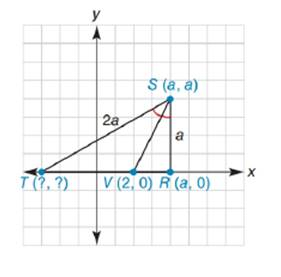Chapter 10.3, Problem 37E### Elementary Geometry for College St...

6th Edition
Daniel C. Alexander + 1 other
ISBN: 9781285195698

#### Solutions

Chapter
Section### Elementary Geometry for College St...

6th Edition
Daniel C. Alexander + 1 other
ISBN: 9781285195698
Textbook Problem
12 views

# In Δ R S T , S V - bisects ∠ R S T . Find the coordinates of point T in terms of a .To determine

To find:

The coordinates of point T in terms of a.

Explanation

The given figure is shown below.

This is solved by angle bisector theorem.

Angle bisector theorem states that an angle bisector of a triangle will divide the opposite side into two segments that are proportional to the other two sides of the triangle.

From the diagram,

TVTS=VRRS

Let the distance between T and V be x.

TS=2a

RS=a

To find VR, use the distance formula.

x1,y1=V2, 0

x2,y2=Ra, 0

Formula for distance between two points

d=x2-x12+y2-y12

Substituting the x and y co-ordinates

d=a-22+0-02

On solving this,

d=a-22+02

d=a-22

Taking square root,

d=a-2

Substitute the values in the theorem statement,

xa-2=2aa

Solving for x,

x=2a·a-2a

Cancelling a,

x=2a·(a2)a

x=2a-4

This is the distance between T and V

### Still sussing out bartleby?

Check out a sample textbook solution.

See a sample solution

#### The Solution to Your Study Problems

Bartleby provides explanations to thousands of textbook problems written by our experts, many with advanced degrees!

Get Started

#### Find more solutions based on key concepts